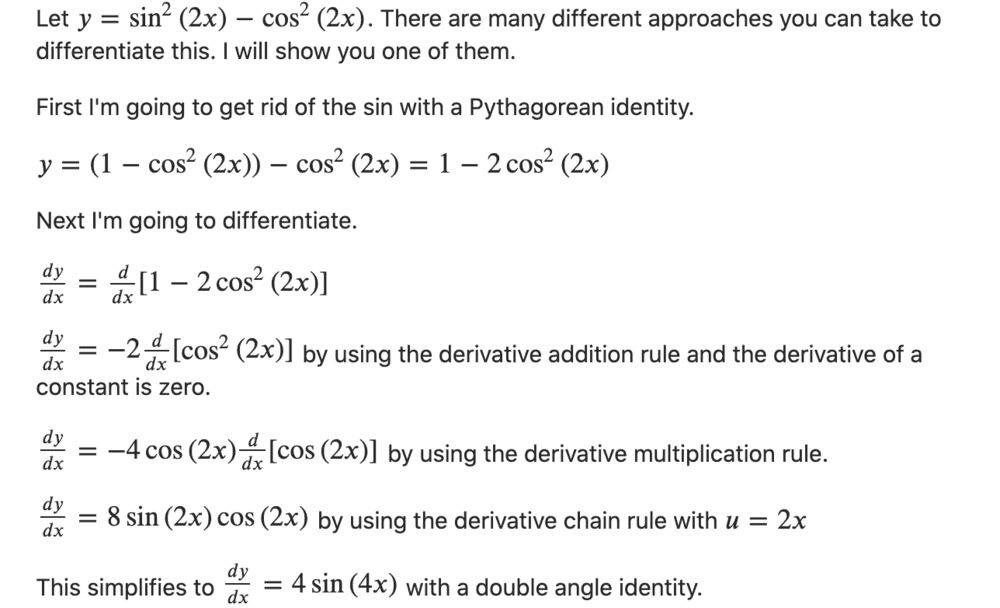# Differentiate and simplify: f(x)=sin^2(2x)-cos^2(2x)

Homework Statement:
Has this been simplified fully?
Relevant Equations:
n/aMark44
Mentor
Homework Statement:: Has this been simplified fully?
Relevant Equations:: n/a

View attachment 262618
Do you have a question here?

benorin
Homework Helper
Gold Member
yes

BvU
Homework Helper
Is that an answer to the quesion in post #1 or the one in post #2 ? Or both ?Let me ask @ttpp1124 : do you expect there exists a simpler expression ? In what way ?

Last edited:
benorin
Homework Helper
Gold Member
yes, it is fully simplified, and of course with trig you could go in many circles with identities but I believe the one stopped at is simplest.

•BvU
pasmith
Homework Helper
I would use $\cos (2\theta) = \cos^2 \theta - \sin^2\theta$, from which immediately $y = -\cos(4x)$.

•BvU and PeroK
BvU